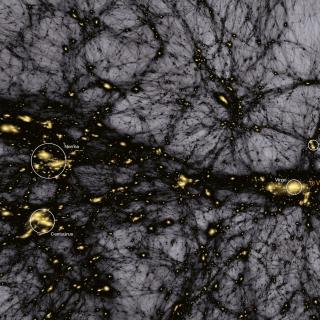# Density weighted angular redshift fluctuations: a new cosmological observable

Hernández-Monteagudo, Carlos; Chaves-Montero, Jonás; Angulo, Raúl E.
Bibliographical reference

Monthly Notices of the Royal Astronomical Society

We propose the use of angular fluctuations in the galaxy redshift field as a new way to extract cosmological information in the Universe. This new probe $\delta z (\hat{\mathbf {n}})$ consists of the statistics of sky maps built by projecting redshifts under a Gaussian window of width σz centred upon a redshift zobs, and weighted by the galaxy density field. We compute the angular power spectrum of the $\delta z (\hat{\mathbf {n}})$ field in both numerical simulations and in linear perturbation theory. From these, we find that the $\delta z (\hat{\mathbf {n}})$ field (i) is sensitive to the underlying density and peculiar velocity fields; (ii) is highly correlated, at the $\gtrsim 60\, {{\ \rm per\ cent}}$ level, to the line-of-sight projected peculiar velocity field; (iii) for narrow windows (σz < 0.03), it is almost completely uncorrelated to the projected galaxy angular density field under the same redshift window; and (iv) it is largely unaffected by multiplicative and additive systematic errors on the observed number of galaxies that are redshift-independent over ∼σz. We conclude that $\delta z (\hat{\mathbf {n}})$ is a simple and robust tomographic measure of the cosmic density and velocity fields, complementary to angular clustering, that will contribute to more complete exploitations of current and upcoming galaxy redshift surveys.